﻿ Project Maths | Learn to use GeoGebra
Working together to improve teaching & learning Leagan Gaeilge
Professional Development ›› Learn to use GeoGebra

## Get started with GeoGebra

To help you get started on using GeoGebra here are some short videos which explain the basics of GeoGebra.

Download GeoGebra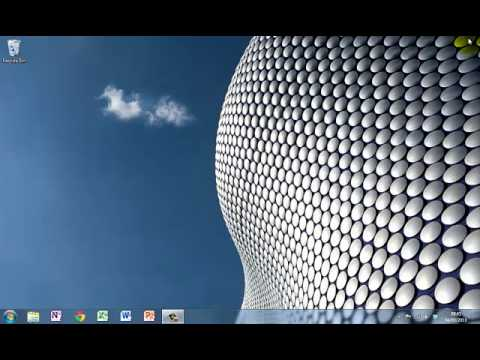Install GeoGebra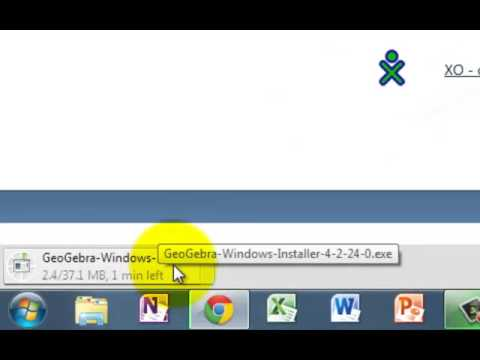Set up GeoGebra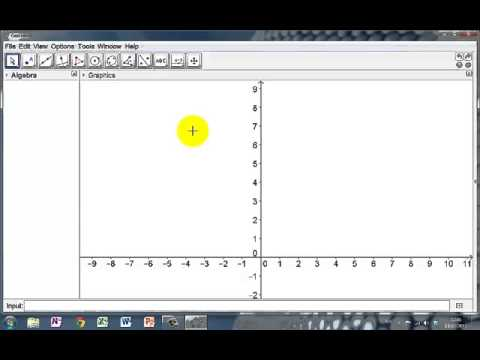This tutorial shows you how to change some basic settings on GeoGebra to make it eas...

Move the graphics view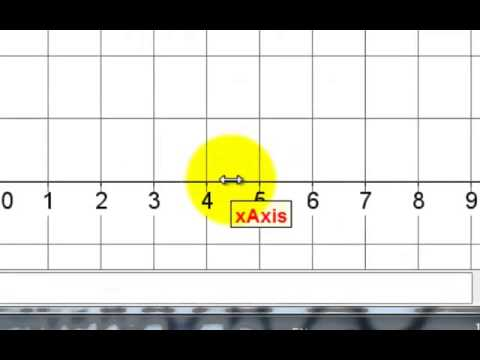Moving and scaling the graphics view is one of the most useful things to know In Geo...

Inserting a picture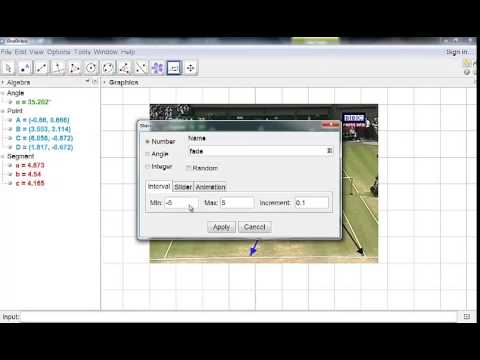When linking maths to real-life it ccan be useful to insert a picture into GeoGebra....

Introduction to geometry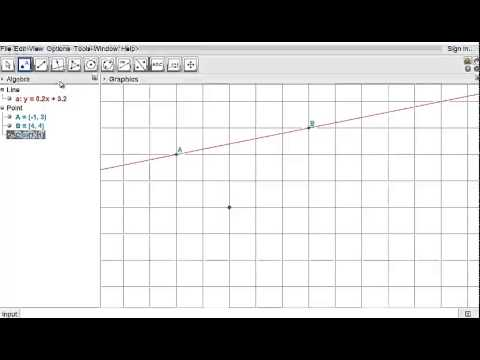Knowledge of a few basic tools makes it easy to teach Geometry using Geogebra. This ...

Bisect a line segment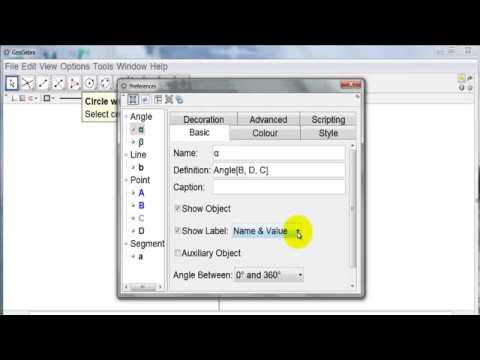Want to construct the perpendicular bisector of a line segment? This tutorial will s...

The equation of a circleUnderstanding the equation of a circle is so much easier when you can see the circle...

Introduction to statistics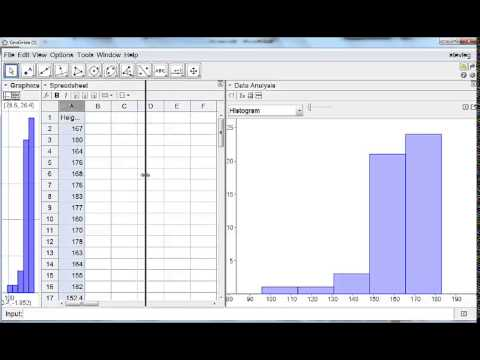Use GeoGebra with Census@SChool data to create charts, calculate means, standard dev...

## What is GeoGebra?

GeoGebra is a free dynamic mathematics software package used by teachers of mathematics in classrooms across the world.  It joins geometry, algebra, tables, graphing, statistics and calculus in one easy-to-use package. It has won several educational software awards across the globe.

## Why do maths teachers and students use GeoGebra?

In its simplest use, GeoGebra allows students to “see” what they are doing with their maths. For example when doing something abstract such as solving a pair of simultaneous equations – it is quick and easy to graph both equations so that students can visualise what the solutions to the equations mean. GeoGebra may be used to give students the opportunity to establish relationships in maths for themselves – for example the fact that a tangent to a circle is always at right angles to the radius at the point of contact. The dynamic nature of GeoGebra makes it easy for students to see how changes in one quantity affect other quantities. For example, when learning about standard deviation or correlation coefficient students can change one item in the data and see what effect this has on each quantity.